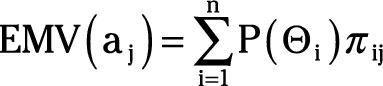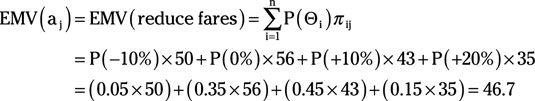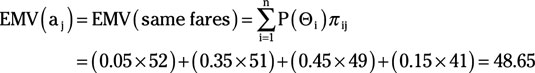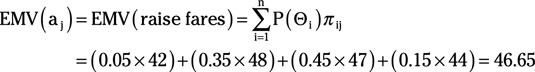##### Managerial Economics For DummiesThe managerial economics theory of maxi-min and mini-max regret criteria for business decisions is all about hoping for the best but expecting the worst. Both of those decision-making criteria focus upon the worst possible outcome, and if you’re like Eeyore, the extremely pessimistic donkey in the Winnie-the-Pooh books, they’re probably the criteria you should use.

The expected monetary value decision-making criterion overcomes the pessimistic approach by incorporating all possible outcomes in the decision-making process. Each state of nature is assigned a probability of occurrence. This probability can be determined from historical data, subjective criteria, or theory. In determining probabilities, however, the sum of the probabilities for all states of nature must equal 1. In other words, you have to specify all possible situations.

After you determine probabilities, calculate the expected monetary value (EMV) of a specific action, aj, through the following formula:This formula indicates that action aj’s expected monetary value equals the summation for all states of nature i = 1 through i = n of the probability of θi occurring, Pi), multiplied by the payoff associated with action aj and state of nature èi, ðij.

After you calculate the expected monetary value, you choose the action with the best expected payoff.

To use the expected monetary value criterion, Global Airlines must determine the probability associated with each state of nature — what happens to oil prices. Currently, Global believes the probability that the price of oil decreases 10 percent is 0.05, the probability that oil prices stay the same is 0.35, the probability that oil prices increase 10 percent is 0.45, and the probability that oil prices increase 20 percent is 0.15.

Note that adding the probabilities for all possible states of nature equals 1.0 (0.05+0.35+0.45+0.15). Thus, Global has considered all possibilities.

To determine the expected monetary value for each action, you take the following steps:

1. Calculate the expected monetary value for reducing fares.

Let reducing fares represent action aj. For each state of nature, multiply the probability of that state of nature by the payoff associated with that state of nature and action aj, reduce fares. Add the resulting values to determine the expected monetary value.2. Calculate the expected monetary value for keeping the same fares.

Let the same fares represent action aj. For each state of nature, multiply the probability of that state of nature by the payoff associated with that state of nature and action aj, same fares. Add the resulting values to determine the expected monetary value.3. Calculate the expected monetary value for raising fares.

Let raising fares represent action aj. For each state of nature, multiply the probability of that state of nature by the payoff associated with that state of nature and action aj, raise fares. Add the resulting values to determine the expected monetary value.4. Choose the action with the highest expected monetary value.

Global Airlines should charge the same fares because its expected monetary value is \$48.65 million. That’s higher than the expected monetary value of reducing fares — \$46.7 million — and the expected monetary value of raising fares — \$46.65 million.

The major advantages associated with the expected monetary value criterion are its inclusion of all possible outcomes and the probability of an outcome occurring. However, although this criterion includes all possible outcomes, it doesn’t take into account an individual’s risk preferences.